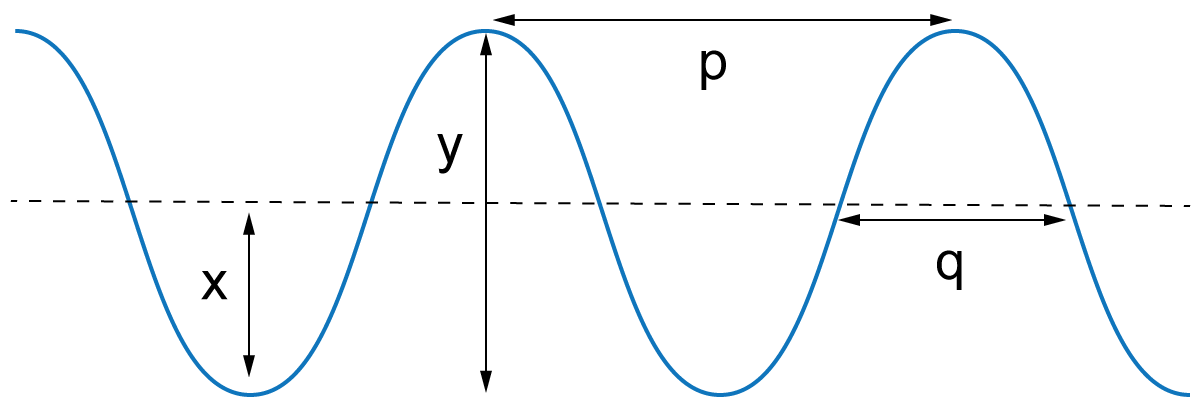OCR GCSE - Waves

page 1

The following diagram shows the electromagnetic spectrum.

1 & 2. What are the 2 missing labels?

 ? transverse waves gamma rays sound waves alpha rays radio waves microwaves Infra red Ultra Violet x rays ? transverse waves gamma rays sound waves alpha rays radio waves

What region of the electromagnetic spectrum ...

3. has the most energy?
4. is used in remote controls for televisions?
5. can be used to kill cancer cells?
6. is used to make florescent inks glow?

Which colour of visible light in the spectrum...

7. Has the longest wavelength?
8, Has the highest frequency?

9. All electromagnetic waves have similar properties. Which one of these is NOT a property of electromagnetic waves?

• A). All travel at the same speed
• B). They all can pass through a vacuum
• C). They can all be reflected
• D). They all have a longer wavelength than sound waves.
10. Which one of these is a longitudinal wave?
• A). Water (surface) waves
• B). Sound wave
• D). X ray wave

11. Which of these is the correct term for a wave where the individual particles move at right angles to the direction of the wave energy transfer?

• A). Transverse
• B). Longitudinal
• C). Refracted
• D). Propagated

12. Which of these arrows correctly shows the amplitude and wavelength?Amplitude Wavelength A). x p B). y p C). x q D). y q